Is it true or false thatandare composite numbers?

# Is it true or false that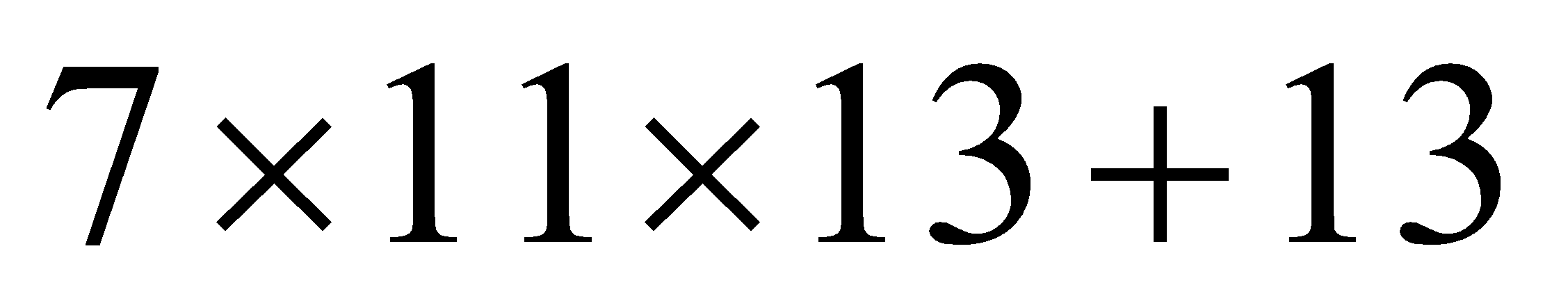and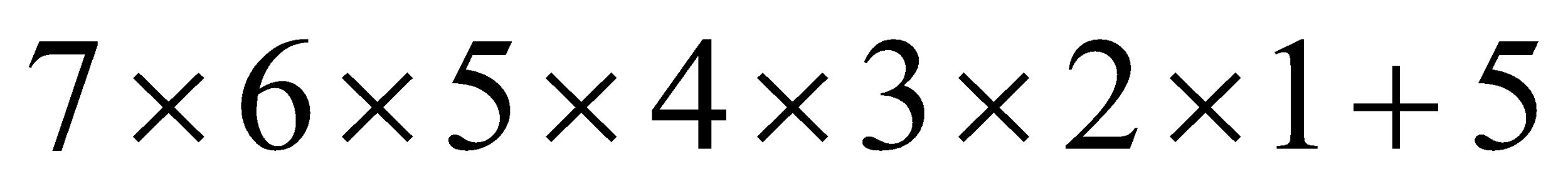are composite numbers?

1. A
True

2. B
False

Fill Out the Form for Expert Academic Guidance!l

+91

Live ClassesBooksTest SeriesSelf Learning

Verify OTP Code (required)

### Solution:

We are given two numbersandand are asked to explain wthether they are composite. Let us consider the first number . We have;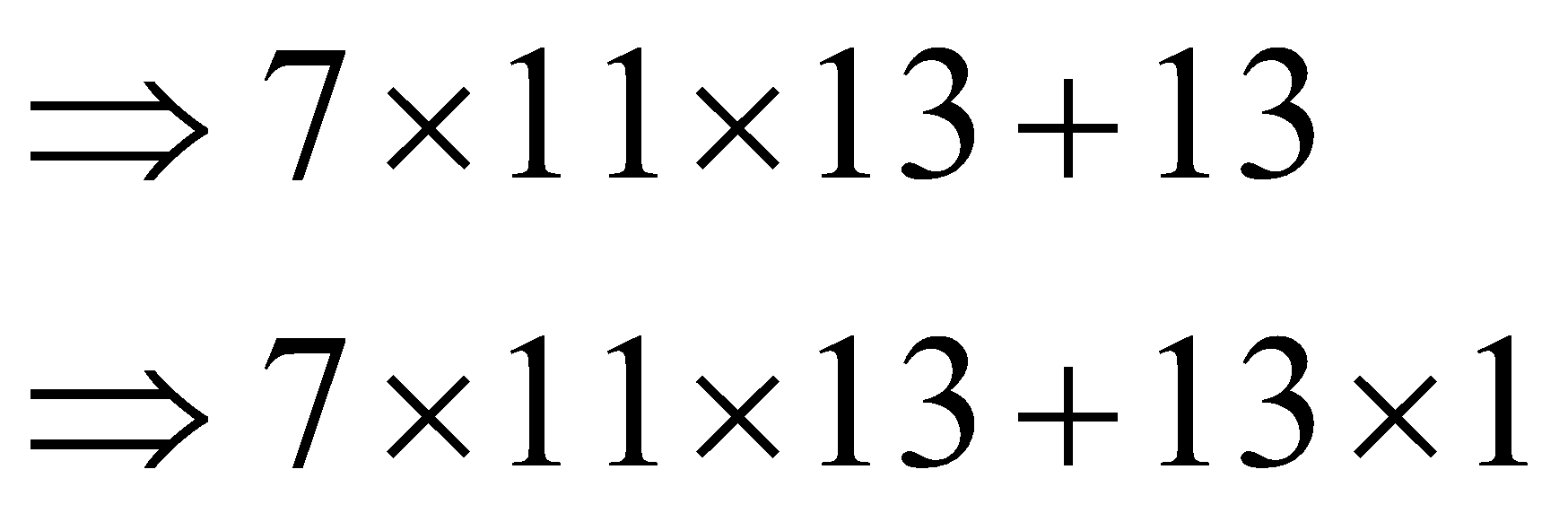We take 13 common in the above step to have;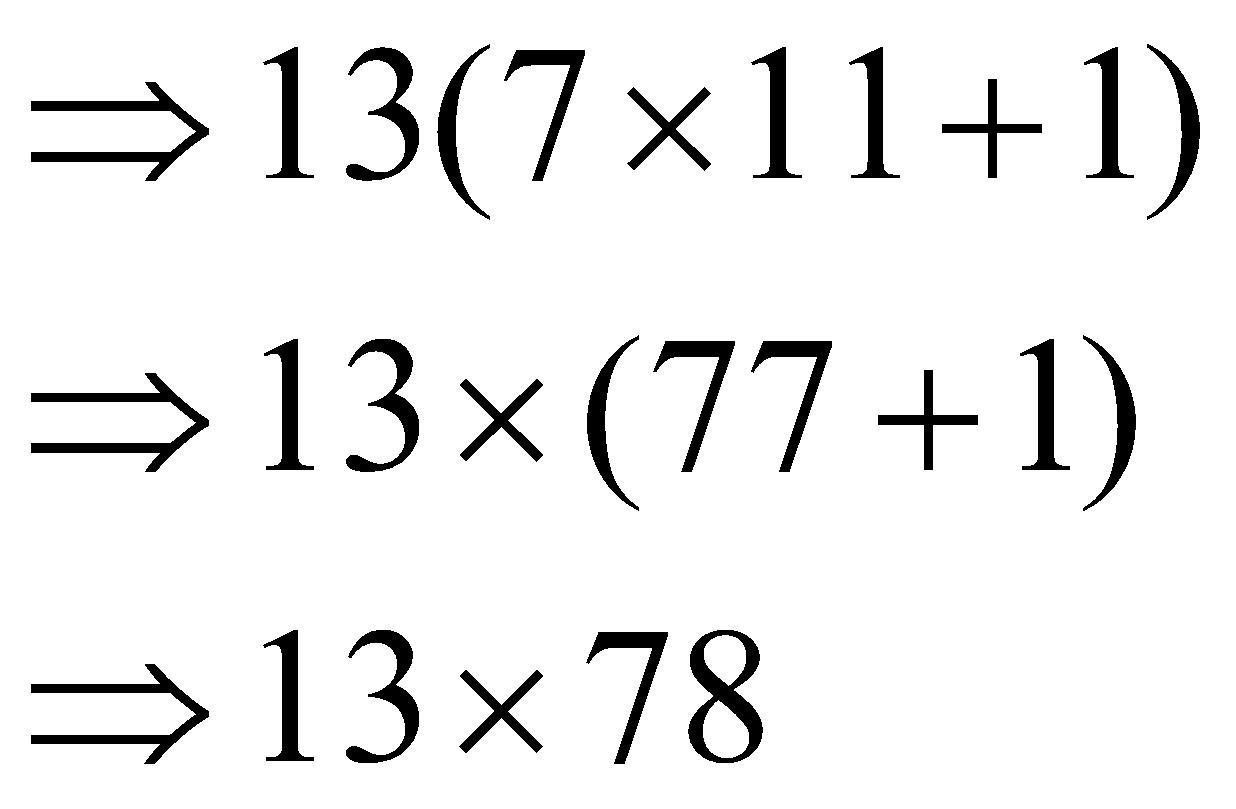We see that except one and the number itself 7×11×13+137×11×13+13 has two more factors 13 and 78. Since now it has more than two factors 7×11×13+137×11×13+13 is a composite number. Let us consider the second number. We have;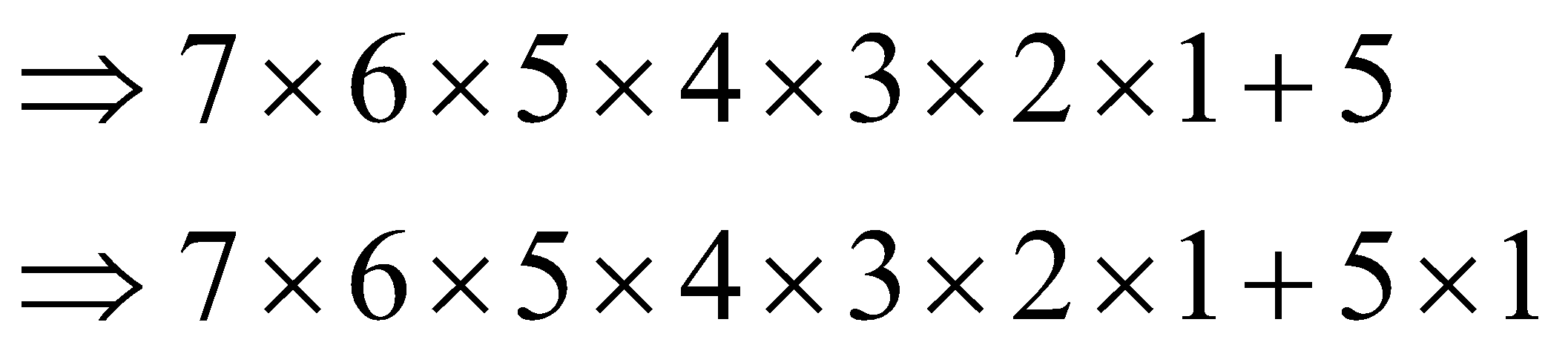We take 5 common in the above step to have;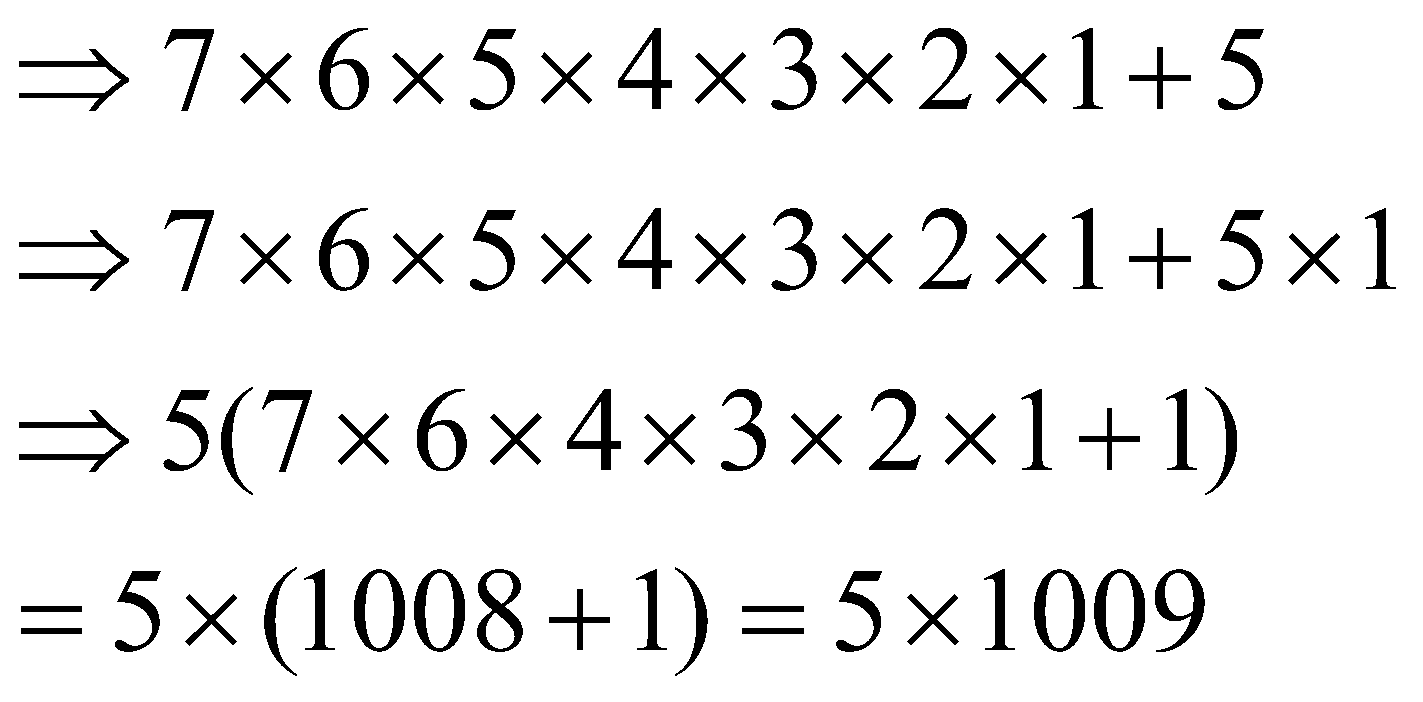So, the statement is true.

## Related content

 Area of Square Area of Isosceles Triangle Pythagoras Theorem Triangle Formula Perimeter of Triangle Formula Area Formulae Volume of Cone Formula Matrices and Determinants_mathematics Critical Points Solved Examples Type of relations_mathematics+91

Live ClassesBooksTest SeriesSelf Learning

Verify OTP Code (required)